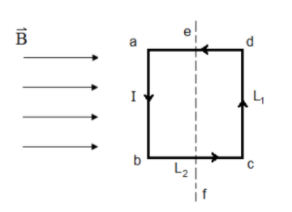# Problem: A rectangular loop with L1 = 0.15 m and L2 = 0.35 m is sitting in a magnetic field B = 0.35 T as shown in the figure. There is a counterclockwise current I = 0.55 A in the loop. Part (a) Which direction does the force on ab act?Part (b) Which direction does the force on cd act?

###### FREE Expert Solution

Part (a)

The direction of i × B is outward.

99% (53 ratings)###### Problem Details

A rectangular loop with L1 = 0.15 m and L2 = 0.35 m is sitting in a magnetic field B = 0.35 T as shown in the figure. There is a counterclockwise current I = 0.55 A in the loop.Part (a) Which direction does the force on ab act?

Part (b) Which direction does the force on cd act?